Next: Doping dependence of the Up: 6.2 Spin-Peierls material CuGeO Previous: Discussion

### Absence of precession in the Zn-doped samples

In the Si-doped system, antiferromagnetic order was clearly exhibited as the spontaneous muon precession (Fig.59). In the Zn-doped systems, Néel order was not clear, although an existence of a static order belowwas presented from the `1/3-component' (Fig.56) and the decoupling behaviors in longitudinal-fields (Fig.58). The absence of muon spin precession suggests that our Zn-doped sample has more randomness than the Si-doped crystal.

One apparent randomness of the Zn-doped samples appeared as the distribution of the Néel temperatures (Fig.57). The spread of, which probably originates from inhomogeneity of the sample, may have smeared out the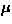SR spectral structures, as discussed in the following.

We may suppose that the spread ofwas caused by an inhomogeneity of the Zn concentration (x). Using the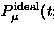phase diagram shown in Fig.53, the distribution of the Néel temperatures (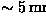) may be mapped to a fluctuation of the Zn concentrations. The result yields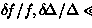and 0.5 % for the x=2, 4 and 8 % systems, respectively. These variations of the Zn concentrations may be mapped to the spread of the field-width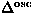using the inset of Fig.57; the result becomes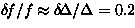and 0.04 for the x=2, 4 and 8 % samples, respectively. The above-mentioned spreads are all Gaussian standard deviation.

TheSR spectrum with the spread of internal fields can be obtained from a convolution:

where, we assumed the ideal muon relaxationto be the relaxation observed in the Si 2% single crystal (eq.59). In the small inhomogeneity limit (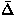), the integral is approximately performed as:

where the precession suffers extra damping as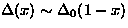, due to the distribution of the frequencies.

In Fig.61, we show a simulatedSR spectrum for the Zn 4% doped system (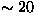), obtained from a numerical integration of eq.60. The precession became less obvious than the Si-doped crystal; this result implies that the macroscopic sample inhomogeneity may be one of the reasons for the absence of muon spin precession in the Zn-doped systems.

The effects of more microscopic randomness, such as substitutions to the spin site with the non-magnetic Zn ions, are not clear at the present stage.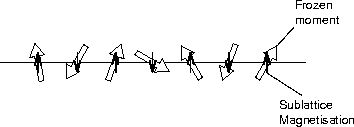Next: Doping dependence of the Up: 6.2 Spin-Peierls material CuGeO Previous: Discussion